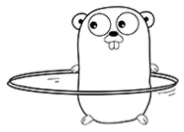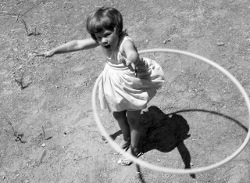# 2 patterns for a do-while loop in Go

yourbasic.org/golangThere is no do-while loop in Go. To emulate the C/Java code

``````do {
work();
} while (condition);``````

you may use a for loop in one of these two ways:

``````for ok := true; ok; ok = condition {
work()
}``````
``````for {
work()
if !condition {
break
}
}``````

### Repeat-until loop

To write a repeat-until loop

``````repeat
work();
until condition;``````

simply change the condition in the code above to its complement:

``````for ok := true; ok; ok = !condition {
work()
}``````
``````for {
work()
if condition {
break
}
}``````